# It's a long way to the top: Solution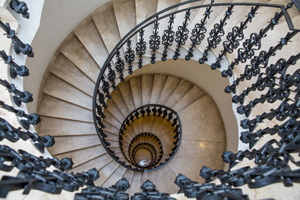Every time I come home I have to climb a flight of stairs. When I'm feeling energetic I sometimes take two steps at a time. This gives me a number of ways to climb the stairs. For example, if there are ten steps, I could climb them taking five leaps of two, giving the pattern

2, 2, 2, 2, 2.

Or I could only use a leap of two at the beginning and the end, giving the patter

2, 1, 1, 1, 1, 1, 1, 2.

How many ways are there all together of climbing the ten steps?

Being a mathematician, I don’t have ten steps of course, but I have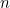steps. Can you find a formula to express the number of ways there of climbingsteps using leaps of one and two?

### Solution

Let’s write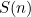for the number of ways to climb a flight of stairs withsteps. There are two possibilities for starting my climb: I could start it using a single step or a leap of two steps. If it’s the former, then there are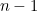steps left to climb, which I can do in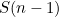ways. If it’s the latter, there are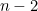steps left to climb, which I can do in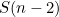ways. This means that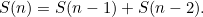This is a recurrence relation. If I know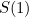and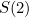then I can work out, which I can then use to work out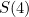, then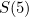, etc.

Clearly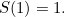There is only one way I can climb up one step and that’s using, well, one step. It’s also easy to see that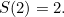I can climb up two steps using two single steps or one leap of two. This gives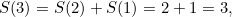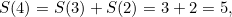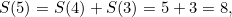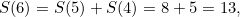and so on. This sequence of numbers might start to look familiar. It's the Fibonacci sequence, which happens to be defined via a recurrence relation in exactly the same way as we defined it here: each number is the sum of the two previous ones. (To be totally accurate, the Fibonacci sequence actually has the first two terms being equal to 1 and 1, rather than 1 and 2, so what we have here is the sequence displaced by a term.)

Carrying on with the calculations we see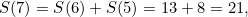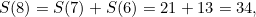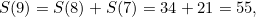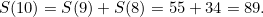So there are 89 ways of climbing a flight of ten steps using steps of one and two!

### The way I look at this is,

Comment

The way I look at this is, the last step taken need not be considered because it is common to every way of climbing the stairs – you always step on the last step. So you only need to look at the number of ways of getting to a position from which you can reach the last step i.e. S(n-2)+S(n-1)

### worked it out different

Comment

when I first tried this problem I tried seeing how many ways there were to go up with a smaller no. of steps. I finally got the formula: 2 to the power of n. So I did 2 to the power of 10 (no. of steps) and got 1024 (no. of ways to go up 10 steps) . Then I checked the solution and it said there were 89 ways to go up 10 steps! I don't understand what I did wrong!? can somebody please explain??

### response

Comment

for 3 steps, the possibilities are 111, 21, and 12. so obviously 2 to the power of n is not a correct formula.

Comment

1111111111
211111111
121111111
112111111
111211111
111121111
111112111
111111211
111111121
111111112
22111111
21211111
21121111
21112111
21111211
21111121
21111112
12211111
12121111
12112111
12111211
12111121
12111112
11221111
11212111
11211211
11211121
11211112
11122111
11121211
11121121
11121112
11112211
11112121
11112112
11111221
11111212
11111122
2221111
2212111
2211211
2211121
2211112
2122111
2121211
2121121
2121112
2112211
2112121
2112112
2111221
2111212
2111122
1222111
1221211
1221121
1221112
1212211
1212121
1212112
1211221
1211212
1211122
1122211
1122121
1122112
1121221
1121212
1121122
1112221
1112212
1112122
1111222
222211
222121
222112
221221
221212
221122
212221
212122
212212
211222
112222
121222
122122
122212
122221
22222
So all of them is 89

### Am I correct tho

Comment

1111111111
211111111
121111111
112111111
111211111
111121111
111112111
111111211
111111121
111111112
22111111
21211111
21121111
21112111
21111211
21111121
21111112
12211111
12121111
12112111
12111211
12111121
12111112
11221111
11212111
11211211
11211121
11211112
11122111
11121211
11121121
11121112
11112211
11112121
11112112
11111221
11111212
11111122
2221111
2212111
2211211
2211121
2211112
2122111
2121211
2121121
2121112
2112211
2112121
2112112
2111221
2111212
2111122
1222111
1221211
1221121
1221112
1212211
1212121
1212112
1211221
1211212
1211122
1122211
1122121
1122112
1121221
1121212
1121122
1112221
1112212
1112122
1111222
222211
222121
222112
221221
221212
221122
212221
212122
212212
211222
112222
121222
122122
122212
122221
22222
So all of them is 89

• Want facts and want them fast? Our Maths in a minute series explores key mathematical concepts in just a few words.